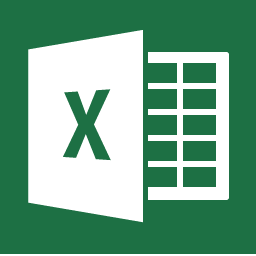# Excel Resources: HomeWhat is Excel?Microsoft Excel is a spreadsheet program included in the Microsoft Office suite of applications. Excel allows users to create, format and manipulate data with formulas. The data is organized in tables of values arranged in rows and columns that can then be manipulated mathematically using both basic and complex arithmetic operations and functions.

Why should you use Excel?

Excel makes manipulating data so easy! With Excel you can...

• Analyze and store data.
• Solve complex math problems.
• Turn your data into charts and graphs.
• Use it to record expenditures and create a budget.
• And so much more!

## More Guides You Might be Interested In

Pivot Tables